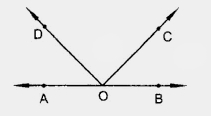# In the figure, write all pairs of adjacent angles and all the linear pairs."

To do:

We have to write all pairs of adjacent angles and all the linear pairs.

Solution:

We know that,

Adjacent angles are two angles that have a common side and a common vertex but do not overlap in any way.

A linear pair can be defined as two adjacent angles that add up to 180 degrees.

From the given figure,

$\angle AOD, \angle COD; \angle BOC, \angle COD; \angle AOD, \angle BOD$ and $\angle AOC, \angle BOC$ are the pairs of adjacent angles.

$\angle AOD, \angle BOD$ and $\angle AOC, \angle BOC$ are the pairs of linear pairs.